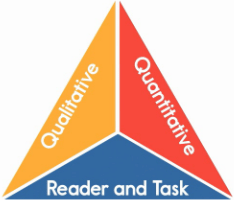# Learning A-Z Text Leveling System

The Learning A-Z Text Leveling System is designed to accurately and reliably measure text complexity to support differentiated instruction. Our leveling system follows the guidelines for determining text complexity as outlined in the Common Core State Standards (CCSS). The Standards call for an evaluation of student reading materials in three areas of text complexity.

## The Common Core Model of Text ComplexityQualitative measures
Qualitative measures are text attributes that can only be evaluated by a human reader. These include factors such as the author's purpose, the levels of meaning, structure of the text, language conventions, language clarity, knowledge demands, and the complexity and importance of visual devices.

The Learning A-Z Text Leveling System takes into account the following qualitative measures:
• Predictability of text
• Text structure and organization
• Logical nature of organization
• Text and feature distractions
• Illustration support
• Infographics
• Complexity
• Text reliance on
• Knowledge demands
• Familiarity of topic (common everyday vs. unfamiliar)
• Single vs. multi-themed
• Intertextual dependence

Quantitative measures
Quantitative measures are statistical measurements of text. These include factors such as average sentence length, number of syllables per word, and the total number of different words.

The Learning A-Z Text Leveling System takes into account the following quantitative measures:
• Total word count
• Number of different words
• Ratio of different words to total words
• Number of high frequency words
• Ratio of high frequency words to total words
• Number of low frequency words
• Ratio of low frequency words to total words
• Sentence length
• Sentence complexity